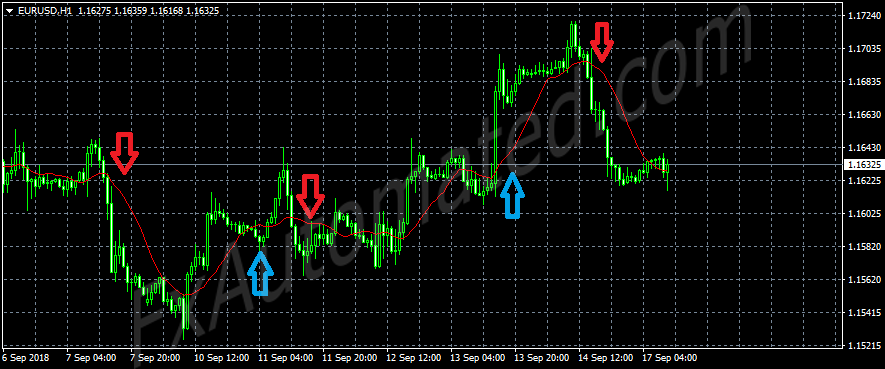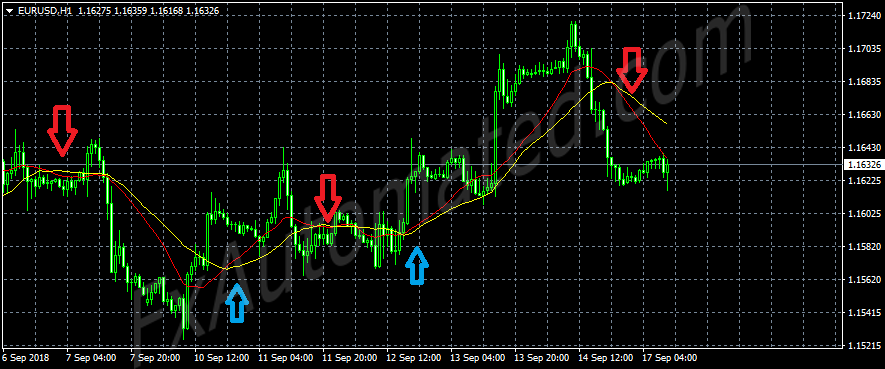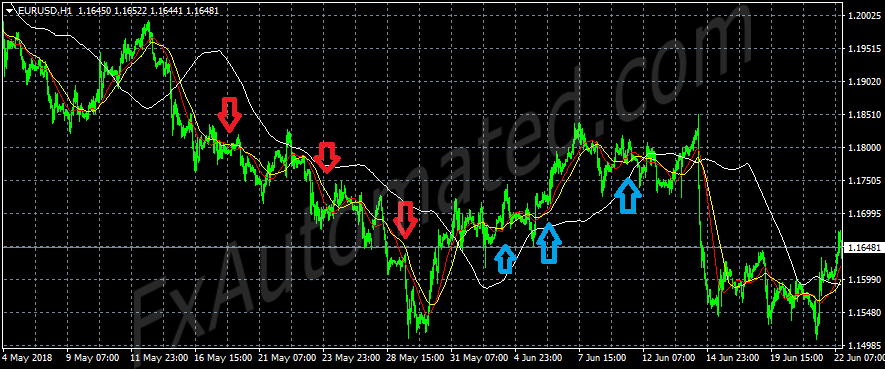# Trading using moving average crosses

The moving average indicator is perhaps the simplest and the first indicator we come across in our trading journeys. Let,s look at several ways it can be used to guide in decision making.

###### Using moving average cross on price as trade signalsThe image above is a candlestick chart with a single Moving average indicator attached to it. We can see that the candlesticks periodically interact with the indicator.

Some of the points where the candlesticks cross the indicator from below to above it, have been marked with a red downward arrow while where the candlesticks cross the indicator from above to below it have been marked with a blue downward arrow. Typically, one would sell when the price crosses the indicator downwards and buy when the price crosses the moving average upwards.

###### Using two moving average crosses to tradeIn the above image, you can see another moving average indicator plotted in yellow color. The blue arrows mark zones where the red MA crosses the yellow one upwards while the red arrows represent zones where the opposite occurs.

This time round we are checking for crosses between the two moving averages instead of the price and the red moving average. In this scenario, the buy signal would be where the red MA crosses the yellow MA upwards while a sell signal would be where the red MA crosses the yellow one downwards.

###### Using three moving averagesAgain, we add another moving average to our chart. Since it’s now a rare scenario to have the three moving averages cross each other at the exact same time, we will have to use the extra moving average plotted in white as a filter of the other two.

This filter MA should be the one with the biggest period and shift setting. Here we would look at the crosses between the red and yellow moving averages and only look for the position of the white moving average.

When the red moving average crosses the yellow one downwards, our white filter MA must be above both the red and the yellow moving averages for us to consider the cross as a sell signal. On the other hand a buy signal is only considered when the red moving average crosses the yellow one upwards while the white moving average is below both the other moving averages. Instead of a third MA, other indicators may also be used together with MA crosses.

###### Downsides of the moving average cross strategy

While the moving average is a very simple indicator to use, it’s also very vulnerable to market conditions. As you may notice, in all the above images, crosses occur more frequently whenever the market is sluggish leaving the moving averages in an intertwined mess. This also means a lot of false signals at this scenario.

Depending on your settings and market scenarios, moving averages can still be used as sure indicators and that’s why they are one of the most popular indicators.